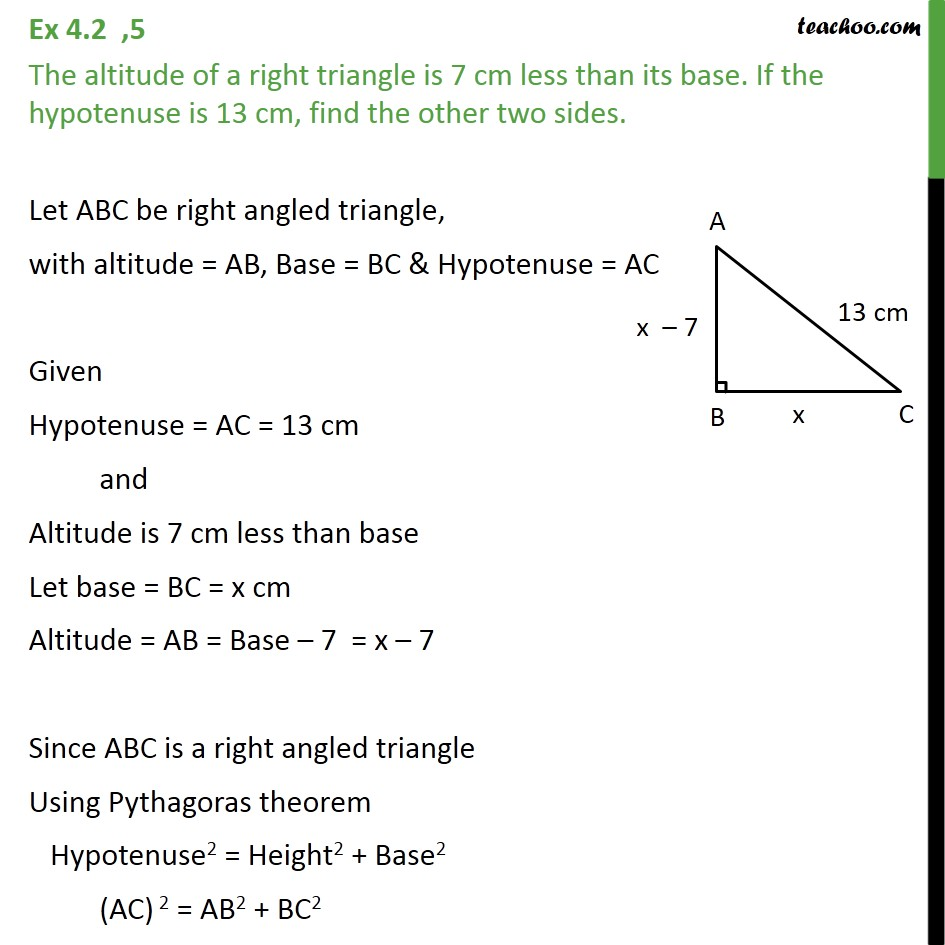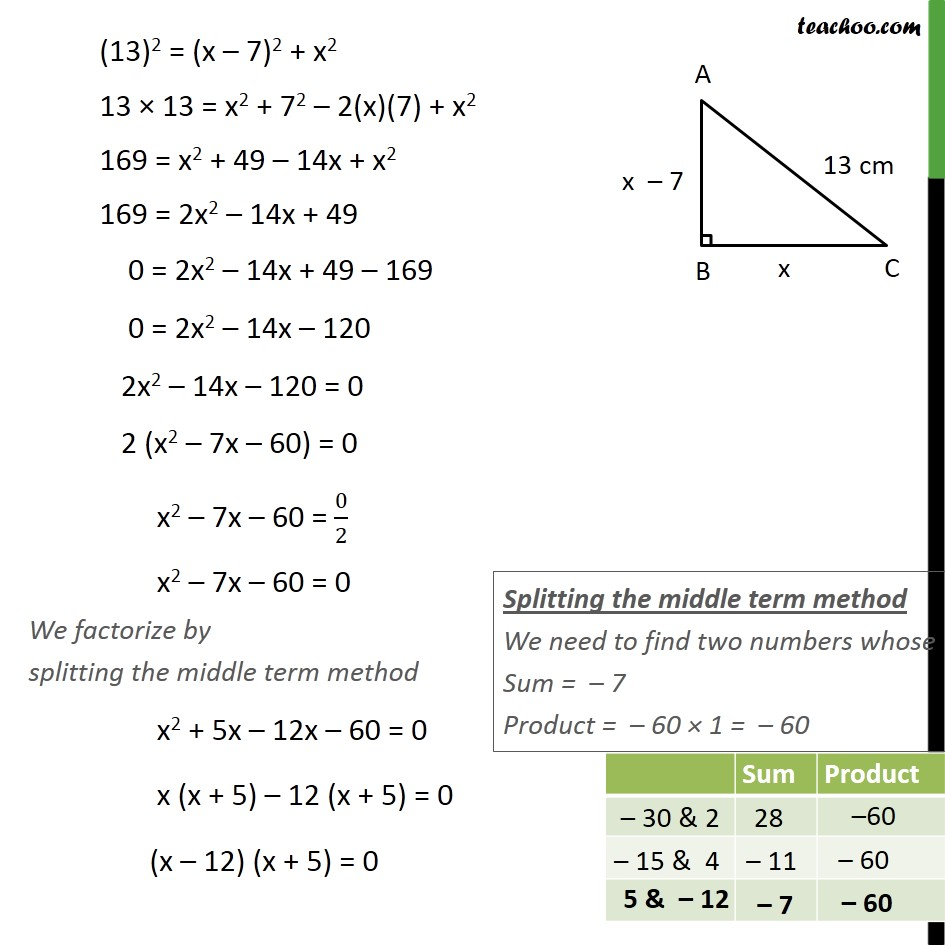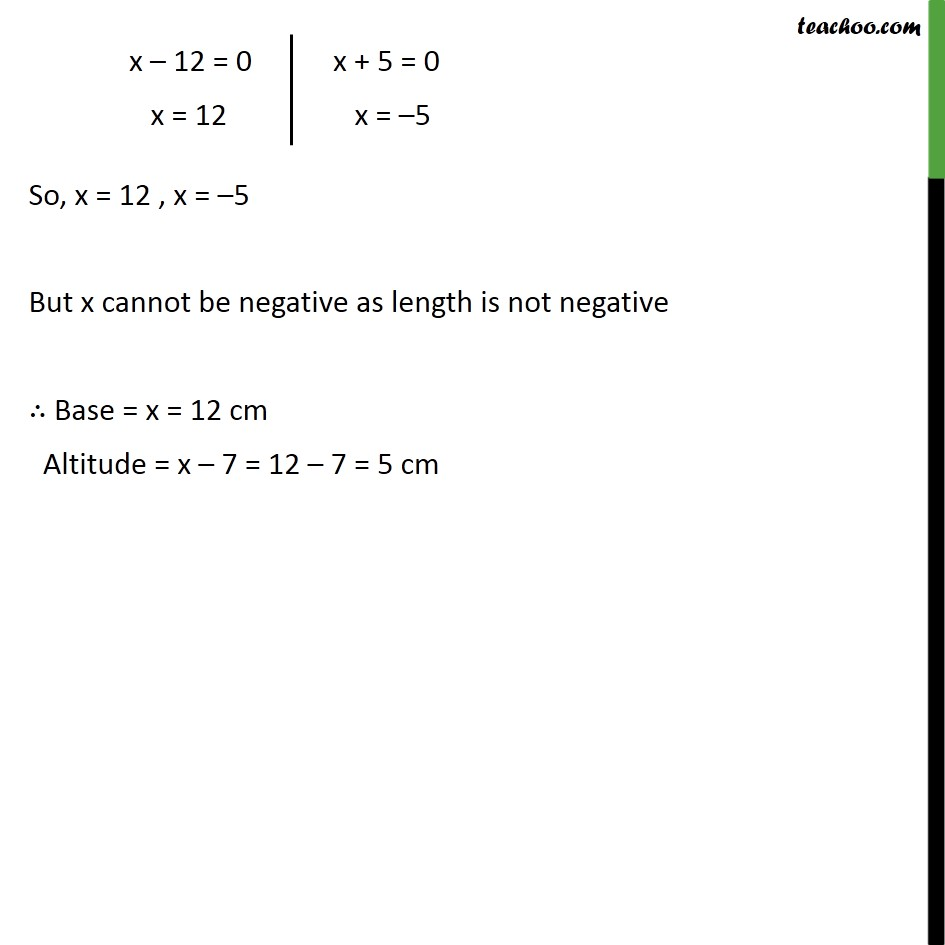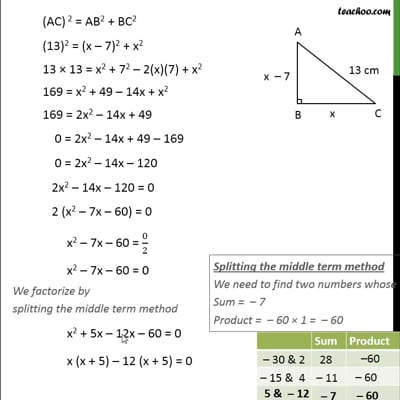Ex 4.2

Chapter 4 Class 10 Quadratic Equations
Serial order wiseThis video is only available for Teachoo black users

Introducing your new favourite teacher - Teachoo Black, at only ₹83 per month

### Transcript

Ex 4.2 ,5 The altitude of a right triangle is 7 cm less than its base. If the hypotenuse is 13 cm, find the other two sides. Let ABC be right angled triangle, with altitude = AB, Base = BC & Hypotenuse = AC Given Hypotenuse = AC = 13 cm and Altitude is 7 cm less than base Let base = BC = x cm Altitude = AB = Base – 7 = x – 7 Since ABC is a right angled triangle Using Pythagoras theorem Hypotenuse2 = Height2 + Base2 (AC) 2 = AB2 + BC2 (13)2 = (x – 7)2 + x2 13 × 13 = x2 + 72 – 2(x)(7) + x2 169 = x2 + 49 – 14x + x2 169 = 2x2 – 14x + 49 0 = 2x2 – 14x + 49 – 169 0 = 2x2 – 14x – 120 2x2 – 14x – 120 = 0 2 (x2 – 7x – 60) = 0 x2 – 7x – 60 = 0/2 x2 – 7x – 60 = 0 We factorize by splitting the middle term method x2 + 5x – 12x – 60 = 0 x (x + 5) – 12 (x + 5) = 0 (x – 12) (x + 5) = 0 So, x = 12 , x = –5 But x cannot be negative as length is not negative ∴ Base = x = 12 cm Altitude = x – 7 = 12 – 7 = 5 cm